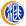# 财经晚餐——你每日必备的交易攻略(5月22日)500)this.width=500 align=center hspace=10 vspace=10 rel=nofollow/>

500)this.width=500 align=center hspace=10 vspace=10 rel=nofollow/500)this.width=500 align=center hspace=10 vspace=10 rel=nofollow/>

500)this.width=500 align=center hspace=10 vspace=10 rel=nofollow/☆欧元债市：意大利公债收益率料创八周来最大周线年期公债收益率本周料将创下八周来最大周线跌幅，受到欧盟复苏基金可能拨款给该国，协助受疫情打击的经济复苏的激励。债券投资人一直期望欧盟能够共同采取行动来解决对抗疫情的成本，而提议中的基金的融资并不会计入已经相当庞大的意大利债务。这项提案协助推动意大利10年期公债收益率本周下跌22个基点，为八周以来最大周线降幅。

500)this.width=500 align=center hspace=10 vspace=10 rel=nofollow/>

500)this.width=500 align=center hspace=10 vspace=10 rel=nofollow/500)this.width=500 align=center hspace=10 vspace=10 rel=nofollow/>

500)this.width=500 align=center hspace=10 vspace=10 rel=nofollow/500)this.width=500 align=center hspace=10 vspace=10 rel=nofollow/>

500)this.width=500 align=center hspace=10 vspace=10 rel=nofollow/500)this.width=500 align=center hspace=10 vspace=10 rel=nofollow/>

500)this.width=500 align=center hspace=10 vspace=10 rel=nofollow/【欧盘必读】风险偏好触发的美元疲弱初步终结？欧银纪要来袭欧元遭遇几大空头施压